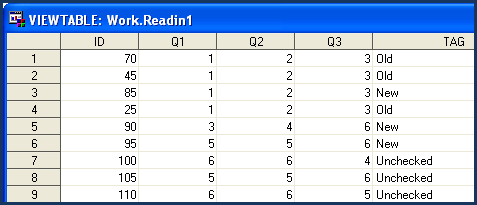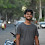# SAS : IF-Then-Else Statements

This tutorial explains how to use IF THEN ELSE statements in SAS, with examples.

Task 1 : Suppose you are asked to exclude some of the observations in a SAS data set from an analysis that you are generating. For example, you want to exclude all IDs whose values are greater than 100.

To accomplish this task, we can use IF, IF-THEN DELETE.

Comparison Operators

 Symbolic Mnemonic Meaning Example = EQ equals IF gender = ‘M’; or IF gender EQ ‘M’; ^= or ~= NE not equal IF salary NE . ; > GT greater than IF salary GT 4500; < LT less than IF salary LT 4500; >= GE greater than or equal IF salary GE 4500; <= LE less than or equal IF salary LE 4500; in IN selecting multiple values IF country IN(‘US’ ’IN’);

1. IF statement

IF (condition is true) => It means subsetting a dataset.

```Data readin;
Input ID Q1-Q3;
cards;
85 1 2 3
90 3 4 6
95 5 5 6
100 6 6 4
105 5 5 6
110 6 6 5
;
IF ID LE 100;
run;
```

The output is shown below :IF ID LE 100 => This would tell SAS to retain only those IDs whose values are less than or equal to 100. In other words, you are removing IDs whose values are greater than or equal to 100.

This can also be done using the IF-THEN DELETE statement.

2. IF-THEN DELETE

IF (condition is true) THEN (delete the selected observations);

```Data readin;
Input ID Q1-Q3;
cards;
85 1 2 3
90 3 4 6
95 5 5 6
100 6 6 4
105 5 5 6
110 6 6 5
;
IF ID GT 100 THEN DELETE;
run;
```

IF ID GT 100 THEN DELETE => This would tell SAS to remove all the IDs whose values are greater than 100.Task 2: Suppose you want to set a tag on all the IDs. The condition is :

If value of ID is less than or equal to 100 set "Old" tag otherwise set "New" tag.

IF (condition is true) THEN (perform this action);
ELSE (perform the action that is set when condition is false);

```Data readin;
Input ID Q1-Q3;
cards;
85 1 2 3
90 3 4 6
95 5 5 6
100 6 6 4
105 5 5 6
110 6 6 5
;
IF ID LE 100 THEN TAG ="Old";
ELSE TAG ="New";
run;
```

Syntax of IF-THEN-ELSE :The output is shown below :The conditions for tagging are as follows :
• If value of ID is less than 75 then TAG = "Old"
• If value of ID is greater than or equal to 75 and less than 100 then TAG = "New"
• If value of ID is greater than or equal to 100 then TAG = "Unchecked"

IF (condition is true) THEN (perform this action);
ELSE IF (perform the action when second condition is true);
ELSE IF (perform the action when third condition is true);

```Data readin;
Input ID Q1-Q3;
cards;
70 1 2 3
45 1 2 3
85 1 2 3
25 1 2 3
90 3 4 6
95 5 5 6
100 6 6 4
105 5 5 6
110 6 6 5
;
length TAG \$20;
IF ID < 75 THEN TAG ="Old";
ELSE IF 75 <= ID < 100 THEN TAG = "New";
ELSE IF ID >= 100 THEN TAG ="Unchecked";
run;
```

Syntax of IF-THEN-ELSE IF :The output is shown below :LOGICAL OPERATORS

 Symbolic Mnemonic Meaning Example & AND Both conditions true IF gender =’M’ and age =1; | OR Either condition true IF gender =’M’ or age =1; ~ or ^ NOT Reverse the statement IF country not IN(‘US’,’IN’);

Task 4: Suppose you want to generate an analysis for Q1 including only responses that are valid (non-missing) and less than 3.

```Data readin;
Input ID Q1-Q3;
cards;
85 1 2 3
90 . 4 6
95 2 5 6
100 6 6 4
105 . 5 6
110 6 6 5
;
IF (Q1 LT 3) AND (Q1 NE .);
run;
```

IF (Q1 LT 3) AND (Q1 NE .) => Since missing values are smaller than any other value, we need to give SAS an additional command to separate out missing values.

The output is shown below:Selecting Multiple Observations :

Suppose you want to set tag "Incorrect" to the specified IDs 1,5,45,76

For this case, the logical statement would look like any one of the following statements. It can be written in three ways shown below.IN Operator

IN operator is used to select multiple values of a variable. It is an awesome alternative to OR operator.
Related Posts
ShareDeepanshu founded ListenData with a simple objective - Make analytics easy to understand and follow. He has over 10 years of experience in data science. During his tenure, he worked with global clients in various domains like Banking, Insurance, Private Equity, Telecom and HR.

12 Responses to "SAS : IF-Then-Else Statements"
1.This comment has been removed by the author.

2.Please can you explain us details about if/then/else.How behind the scene it works. I try to get the clue but still I am not clear.For example putting if only without else how it works ? putting else if how it works ? At end only else how it works ? Putting only if without then how it works? How it works with do loop and array ? Basically while I am trying to understand Sas certified question (Mostly program with if/then/else/do statement and question is how many observations)it making me a lot confuse.

1.Are you from a non-technical background?

3.This comment has been removed by the author.

4.Hi. It shows me LE instead GE in below code

length TAG \$20;
IF ID < 75 THEN TAG ="Old";
ELSE IF 75 <= ID < 100 THEN TAG = "New";
ELSE IF ID >= 100 THEN TAG ="Unchecked";
run;

ELSE IF 75 <= ID < 100 THEN TAG = "New";

5.It says if ID is less than or equals to 75 or less than 75.
It means if ID is between 75 and 100 then tag as New.

6.length Tag \$30;
If ID in (1,5,45,76) then tag ='Incorrect';
run;
proc print;run;

What's wrong in my code?
unable to show as incorrect in the output

1.You didn't specify what data to print.

run;

2.ID does not contain values (1,5,45,76).

3.length Tag \$30;
If ID in (1,5,45,76) then tag ='Incorrect';
run;
proc print;run;

What's wrong in my code?
unable to show as incorrect in the output

Ans: it will not show incorrect in output because the id's which you mentioned in in (1,5,45,76)statement are not present in sas dataset. so change the values as we have in readin dataset and try it will work.

7.Hii Sir,
• Create a new SAS data set, work.shoerange.
• Create a new character variable SalesRange that will be used to categorize the observations into
three groups.
• Set the value of SalesRange to the following:
o Lower when Sales are less than \$100,000.
o Middle when Sales are between \$100,000 and \$200,000, inclusively.
o Upper when Sales are above \$200,000.

RUN this

1.DATA SHOERANGE;
LENGTH SHOERANGE\$10.;
SET SASHELP.SHOES;
IF SALES < 100000 THEN SHOERANGE ="LOWER";
ELSE IF (SALES GT 10000) OR (SALES LT 20000) THEN SHOERANGE ="MIDDLE";
ELSE IF SALES > 200000 THEN SHOERANGE="UPPER";
RUN;

Next → ← Prev